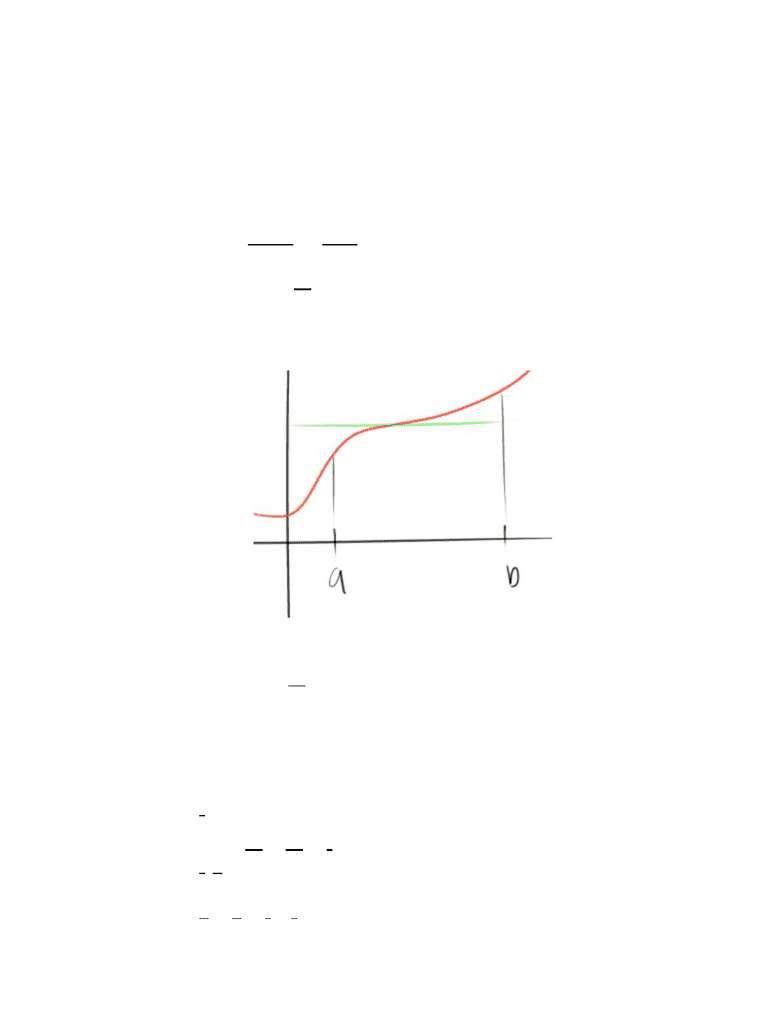Study Guides (390,000)
US (220,000)
UC-Irvine (3,000)
MATH (600)
MATH 2B (100)
All (60)
Midterm

# MATH 2B Study Guide - Fall 2018 Midterm Notes - Tanx, UduPremium

Department
Mathematics
Course Code
MATH 2B
Professor
All
Study Guide
Midterm

This preview shows pages 1-2. to view the full 6 pages of the document.Think about the equation for average speed
The average speed is the average speed you are at in the time that you traverse
a certain distance
vg. Speed A= ba
s(b) − s(a)=time
distance
We can ignore the numerator because it is a constant
vg. Speed [s(x)] (between a and b)A= 1
ba
(x) V(x)S=
The average velocity is average of all the y-values
The official formula
vg. value (t)dta = 1
ba
b
a
V
Example #4
Find the average value of (x) on [0, ]f=x24
dx
3
1
4
1
x2
1
ba= 1
4−1 = 3
1
( ) (between 1 and 4)
3
13
x3
Take the integral
9
43 9
13= 9
3=3
1
Plug in values
Average Value
Section 6.5

Only pages 1-2 are available for preview. Some parts have been intentionally blurred.

Subscribers OnlyExample #5
Given and , what is C?(x) x x Cx 5f= 3− 2 2+ + (x) has an average of 2 on [0, ]f1
x x
1
ba
1
0
x3− 2 2+C+ 5
Take the integral
(x x) (between 0 and 1)
1
14
x43
2 3 +2
Cx2+ 5
Plug in values
) (0)(4
13
2+2
C+ 5
Then simplify
2 = 12
6C + 55
4 55 6C2 =
C=6
−31
Example #6
(x) x on [0, ]f= p1
Average value of 3
2
What is ?p
vg dxa = 1
1−0
1
0
xp
Compile the integral
(between 0 and 1)
p+1
xp+1
Take the integral
1
p+2 = 3
2
Plug in values
2(p)3 = + 2
Simplify
2p1 =
p= 2
1